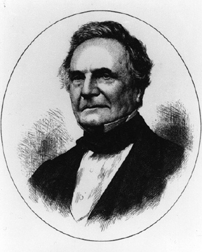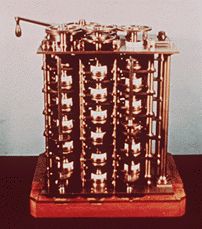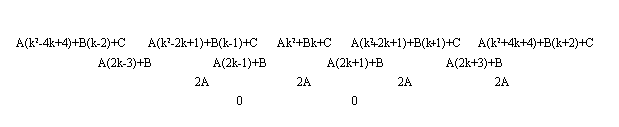# Divided Differences

##### Age 16 to 18

Published 2002 Revised 2016

# 1 A first look at differencing

When in 1821 the English mathematician Charles Babbage invented what we now recognise as the grandfather of the modern computer he called it the 'Difference Engine' since it was intended to take over the work of making mathematical tables by the techniques described in this article.Photo courtesy of the Charles Babbage Institute, University of Minnesota, Minneapolis. Reproduced by courtesy of IBM Computer Museum.
Today every engineer, mathematician or physicist has access to their own pocket calculator and personal computer. But there were engineers, mathematicians and physicists long before electronic computers existed. How did they perform the long and complicated calculations that their trades demand?

Part of the answer lay in the the use of 'tables'. Nowadays if we wish to know the value of $\sin 44^{\circ}$ we press the correct button on our calculator. In the past we took down a book of tables' and looked it up. As a simple example here is a table of squares.

 n 0 1 2 3 4 5 6 7 8 9 n2 0 1 4 9 16 25 36 49 64 81

If we want to know 52 we simply look at the entry under 5.

Exercise 1 Produce a similar table of cubes.

Mathematicians found a large number of tricks to make the construction and use of tables easier. Here is one of them. Consider our table of squares. Let us take the difference between successive entries as shown below.

 0 1 4 9 16 25 36 49 64 81 1 3 5 7 9 11 13 15 17

Now take the difference of successive differences to obtain

 0 1 4 9 16 25 36 49 64 81 1 3 5 7 9 11 13 15 17 2 2 2 2 2 2 2 2 2

Finally we take the difference of the differences of the differences to obtain

 0 1 4 9 16 25 36 49 64 81 1 3 5 7 9 11 13 15 17 2 2 2 2 2 2 2 2 2 0 0 0 0 0 0 0 0

Let us try the same thing on a table of 4th powers.

 n 0 1 2 3 4 5 6 7 n4 0 1 16 81 256 625 1296 2401

This time successive differencing produces the following table.

 0 1 16 81 256 625 1296 2401 1 15 65 175 369 671 1105 14 50 110 194 302 434 36 60 84 108 132 24 24 24 24 0 0 0

Exercise 2 (i) Extend the table of 4th powers n4 to cover n=8 and n=9. Verify that the pattern suggested above continues to hold.
(ii) Find the effect of repeated differencing on your table of cubes.

Exercise 3 (i) Try the effect of repeated differencing on 3n3 -5n2 .
(ii) Try the effect of repeated differencing on a polynomial of your choice.

It very much looks as though the effect of repeated differencing a polynomial of degree m is to produce a row of zeros after at most m+1 differences. Let us try our conjecture on the most general quadratic f(n)=An2 +Bn+C. Here is part of the initial table

 n k-2 k-1 k k+1 k+2 f(n) A(k2 -4k+4)+B(k-2)+C A(k2 -2k+1)+B(k-1)+C Ak2 +Bk+C A(k2 +2k+1)+B(k+1)+C A(k2 +4k+4)+B(k+2)+C

and here are the repeated differencesSince we could pick any k to investigate, we have verified the conjecture for the general quadratic.

Exercise 4 Verify the conjecture for the general cubic.

The next exercise requires more algebra.

Exercise 5 (i) If f(n)=nk show that f(n+1)-f(n) is a polynomial in n of degree k-1.
(ii) If g(n) is a polynomial of degree k show that g(n+1)-g(n) is a polynomial in n of degree k-1.
(iii) Prove our conjecture that the effect of repeated differencing a polynomial of degree m is to produce a row of zeros after at most m+1 differences.

We now observe that we can reconstruct the whole of a table of differences for a polynomial from a small part of it. For example, suppose we are told that f is a quadratic polynomial and its table of differences looks like

 ? ? ? 7 ? ? ? ? ? ? ? ? ? 6 ? ? ? ? ? ? ? ? 2 ? ? ? ? ? 0 0 0 0 0 0 0 0

We can immediately fill in the last but one row to obtain

 ? ? ? 7 ? ? ? ? ? ? ? ? ? 6 ? ? ? ? ? 2 2 2 2 2 2 2 2 2 0 0 0 0 0 0 0 0

and then the next to obtain

 ? ? ? 7 ? ? ? ? ? ? 0 2 4 6 8 10 12 14 16 2 2 2 2 2 2 2 2 2 0 0 0 0 0 0 0 0

Exercise 6 (i) Fill in the top row and check that we have the table of n2 -n+1 with the leftmost entry corresponding to n=0.
(ii) Fill in in the following table of differences for a quadratic.

 ? ? ? 9 ? ? ? ? ? ? ? ? ? 16 ? ? ? ? ? ? ? ? 6 ? ? ? ? ? 0 0 0 0 0 0 0 0

Check that the table you get is for f(n)=3n2 -5n-3 with the leftmost entry corresponding to n=0.

Now suppose that we want to tabulate f(n)=n3 -3n2 +5n+1. Here is a partial tabulation which the reader should check.

 n -4 -3 -2 -1 0 1 2 3 4 5 f(n) ? ? -29 -8 1 4 7 ? ? ?

We can now draw up a partial table of differences which the reader should check.

 ? ? -29 -8 1 4 7 ? ? ? ? ? 21 9 3 3 ? ? ? ? ? -12 -6 0 ? ? ? ? ? 6 6 ? ? ? ? ? 0 ? ? ?

But we can now reconstruct the full table of differences. Here is a partial reconstruction

 ? -54 -29 -8 1 4 7 16 ? ? ? 39 21 9 3 3 9 ? ? ? -18 -12 -6 0 6 ? ? ? 6 6 6 6 ? ? ? 0 0 0 ? ?

Notice that we have extended our original table to read

 n -4 -3 -2 -1 0 1 2 3 4 5 f(n) ? -54 -29 -8 1 4 7 16 ? ?

Exercise 7 Continue the reconstruction of the table of differences to the point where you have the value of f(n) for n=-4, n=4 and n=5. Check your answers by computing f(-4), f(4) and f(5) directly from the definition.

Exercise 8

Let $f(n)=3n^2+2n+1$. Compute f(n) for n=-2, -1, 0, 1, 2. Form the associated partial table of differences and then reconstruct the table of differences sufficiently far that you have f(n) for all n with $-5\leq n\leq 4$. Check your answer by computing f(n) directly.

The fact that (at least for polynomials) we can use differences to reconstruct a full table from a partial table gives a strong hint as to why table makers were so interested in these ideas.

Here is another reason. Suppose we have a table like the following.

 0 0 0 0 0 1 0 0 0 0 0

in which all the entries are zero except one. If we compute successive differences we get

 0 0 0 0 0 1 0 0 0 0 0 0 0 0 0 +1 -1 0 0 0 0 0 0 0 1 -2 1 0 0 0 0 0 1 -3 3 1 0 0 0 1 -4 6 -4 1 0

Exercise 9 (i) Calculate one further row of differences.
(ii) Conjecture the form of the nth row of differences.
(iii) [Harder and optional] Prove your conjecture.

Exercise 10 (i) Find the successive differences for the table

 0 0 0 0 0 A 0 0 0 0 0

(ii) Find the successive differences for

 13 7 3 1 1 3 7 13 21

(iii) Find the successive differences for

 13 7 3 1 1 3 (7+A) 13 21

(iv) Suppose f is a cubic polynomial. What is the fifth line in the table of successive difference for f? Find the fifth line of the table of successive differences whose first line is

 f(n) f(n+1) f(n+2) f(n+3) f(n+4) f(n+5)+A f(n+6) f(n+7) f(n+8) f(n+9) f(n+10)

Explain how you can find A just by looking at the 5th line.
(v) The following table is supposed to represent the values of a quadratic but I have made a mistake in one entry.

 n -1 0 1 2 3 4 5 6 7 8 9 f(n) 8 3 0 -1 0 5 8 15 24 35 49

By successive differencing find the error and correct it.
(vi) Explain how you can locate and correct a single error in a table of a polynomial of degree k.
(vii) Explain how you would try to locate and correct a single error in a table of a polynomial whose degree you did not know.

Successive differencing thus gives an excellent way of finding and correcting errors in tables.

# 2 A second look at differencing

So far we have only looked at tables of a function f as a method of finding f(n) where n is an integer. However we really want to know f(x) at all points x. In other words, knowing f(0), f(1),..., f(m) we want to find f(x). We shall see that this can be done but we will need to raise the mathematical level a little bit.

The key is a set of observations which go back at least as far as Newton.

Exercise 11 (i) Consider the function f2 (x)=x(x-1)/2!. We tabulate it as follows.

 n 0 1 2 3 4 5 6 7 8 9 f(n) 0 0 1 3 6 10 15 21 28 36

Find the table of successive differences.
(ii) Do the same for f3 (x)=x(x-1)(x-2)/3!, f4 (x)=x(x-1)(x-2)(x-3)/4!. Do the same for f1 (x)=x/1!=x and f0 (x)=1.
(iii) Conjecture the general pattern.
(iv) [Harder and optional] Prove your conjecture.

Exercise 12 (i) Let f(x)=Af3 (x)+Bf2 (x)+Cf1 (x)+D. Find the table of successive differences as in the previous exercise. If we take it in the form

 f(0) f(1) f(2) f(3) f(4) f(5) f(6) f(7) f(8) f(9) ? ? ? ? ? ? ? ? ? ? ? ? ? ? ? ? ? ? ? ? ? ? ? ? ? ? ? ? ? ? ? ? ? ? ?

identify ?, ?, ? and ?.
(ii) If g is any cubic, show that we can find a, b, c and d such that

g(x)=af3 (x)+bf2 (x)+cf1 (x)+d.

Hence show that g can be found from its table of successive differences.
(iii) Conjecture the general pattern.
(iv) [Harder and optional] Prove your conjecture.

We can extend the results of the last exercises a bit. Suppose that f is a polynomial of degree m. If we form the the following table of differences (here h> 0)

 f(a) f(a+h) f(a+2h) f(a+3h) f(a+4h) f(a+5h) f(a+6h) f(a+7h) f(a+8h) ... 0 ? ? ? ? ? ? ? ... ?1 ? ? ? ? ? ? ... ?2 ? ? ? ? ? ... ?3 ? ? ? ? ... ?4 ? ? ? ...

and so on, then

 $$f(a+k)=\alpha_{0}+\alpha_{1}\frac{k}{h}+ \alpha_{2}\frac{k(k-h)}{2!h^{2}}+ \alpha_{3}\frac{k(k-h)(k-2h)}{3!h^{3}}+\dots$$ *

Exercise 13 [Optional] Prove this.

If we use formula *

for $0\leq k\leq mh$

then we say that we are interpolating . If we use formula * for k outside this region we say that we are extrapolating .

The sceptical reader may have noticed that, although we began our discussion by talking about tables of the sine function everything that followed dealt with polynomials. However, as the mathematicians of the 17th century discovered, the kind of nice' functions that we wish to tabulate look very much like polynomials over small ranges of values. Since they look like polynomials, formula * which applies to polynomials will apply to them (to a very close approximation). We need only calculate our desired function at a small number of values and then we can use equation * to find its values at other points.

For example, here are the values of ln x at a certain number of values.

 x 5 5.1 5.2 5.3 5.4 f(x) 1.60944 1.62924 1.64866 1.66771 1.6864

We obtain the following table of differences.

 1.60944 1.62924 1.64866 1.66771 1.68640 0.01980 0.01942 0.01905 0.01869 -0.00038 -0.00037 -0.0036 0.00001 0.00001

Thus, in equation * , ?0 =1.60944, ?1 =0.01980, ?2 =-0.00038 and ?4 =0.0001. We thus hope that

$\ln(5+k)\approx1.60944+0.01980\frac{k}{h}+ -0.00019\frac{k(k-h)}{h^{2}}+ +0.00001\frac{k(k-h)(k-2h)}{6h^{3}}.$

Trying this with $k=0.11$ gives $\ln 5.11\approx 1.63120$

and this turns out to be correct to the number of digits given.

Exercise 14 Compute ln 5.16 using our approximation. Compare this with the correct answer. Repeat the exercise for ln y where you choose y with

$5 < y < 5.4$.

Although functions like ln behave like polynomials over small ranges of values, they need not behave like polynomials over large ranges. It is thus not surprising that an idea which works well for interpolating (that is, trying to find the value of a function f at a point using values of f at points close by) works less well (or fails entirely) when we try to use it to guess the value of a function at points far away from those where we know its value.

Exercise 15 Compute ln 10 using our approximation. Compare this with the correct answer.

There is a much more serious problem associated with differencing which I have avoided mentioning up to now. So far, I have assumed that all the initial tabular values are given exactly. In reality, we usually work to a certain number of decimal places. Suppose that we round to the nearest integer. Then a table which looks like

 0 0 0 0 0 0 0 0

with a table of differences

 0 0 0 0 0 0 0 0 0 0 0 0 0 0 0 0 0 0 0 0 0 0 0 0 0 0 0 0 0 0

could actually represent

${ {1 \over 2} -{1 \over 2}{1 \over 2} -{1 \over 2}{1 \over 2} -{1 \over 2}{1 \over 2} -{1 \over 2} }$

with a table of differences

 1/2 -1/2 1/2 -1/2 1/2 -1/2 1/2 -1/2 1 -1 1 -1 1 -1 1 -2 2 -2 2 -2 2 4 -4 4 -4 4 -8 8 -8 8

Thus if the first line of a table of differences is only known to an accuracy of epsilon; the second line is only known to an accuracy of 2 epsilon; and the nth line to an accuracy of 2n epsilon;.

In practice this means that, initially, the entries in successive lines of a table of differences will tend to decrease (just as we saw when we used exact arithmetic) but because the errors are increasing there will come a point when the errors swamp the calculation and the entries in successive lines will tend to increase.

As an example consider the entries in a table of sines (x represents degrees).

 x 10 11 12 13 14 15 16 17 sin x 0.1736 0.1908 0.2079 0.225 0.2419 0.2588 0.2756 0.2924

Here is the table of differences

 0.1736 0.1908 0.2079 0.2250 0.2419 0.2588 0.2756 0.2924 0.0172 0.0171 0.171 0.169 0.0169 0.168 0.0168 -0.0001 0.0000 -0.0002 0.0000 -0.0001 0.0000 0.0001 -0.0001 -0.0002 0.0002 -0.0001 0.0001 -0.0002 -0.0001 0.0004 -0.0003 0.0002 -0.0001 0.0005 -0.0007 0.0005 0.0006 -0.0012 0.0012

It is clear that only the first two lines of the table of differences (and perhaps, if we think hard, the third) carry any information. In the remaining lines the `noise' of the errors drowns out everything else.

This means that when we interpolate we must confine ourselves to using the first two lines and our version of * will read

$\sin (10+k)\approx0.1736+0.0172k.$

(However, as the reader can verify, this remains a pretty accurate formula for $k$ with $0\leq k\leq 1$.)

Exercise16 Choose a table (or make your own) of some function, form the table of differences, note the line at which error noise becomes dominant. Use the part of the table above that line to interpolate at some point. Check the accuracy of your interpolation. It is very instructive to investigate how the number of significant figures and the spacing of the points of your table affect the result.

We can now see one way in which we can compile tables of a function f like ln or sin with a given level of accuracy. We compute f at a relatively small number of points to much higher accuracy than the table demands. (Of course, these calculations may be quite lengthy but we only need do a few of them.) We obtain the values at f at the remaining points by easy interpolation. Notice that once we have obtained a few points using * we can the build up the rest of the table using the techniques of Exercise 16.

For four hundred years science and technology depended on tables. Books of tables made it possible to find the orbits of the planets, to navigate the globe, and to make an industrial revolution. The book of logarithms was as much a symbol of science as the microscope. Yet, even then, few people bothered to celebrate the labours of the maker of tables. Nowadays their work is completely forgotten. I hope that this essay may help the reader appreciate the work of these unsung heros.

Dr Tom KÃ¶rner is a Reader in the Department of Pure Mathematics and Mathematical Statistics at the University of Cambridge and a Fellow of Trinity Hall.